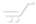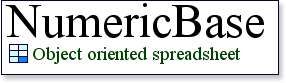# Formula terms

Terms and operators.
Formulas in NumericBase follow a specific syntax, which includes "terms" that are combined together using "operators" to form the formula.

The different terms are "constant", "name", and "function call". These are outlined below.

Constant.
Can be one of the following:
1. Null constant. Represented by the question mark character (?). This constant is most useful in range formulas.
2. String constant. Any combination of characters that is surrounded by quotes. For example:
• "red"
• "3434"
• "any combination including special characters such as \$,%,^,&, etc.."
3. Numeric constant. Any combination of digits with at most one decimal points, and at most one exponent. For example:
• 45 - have no decimal point and no exponent.
• 4.534 - have one decimal point,
• 4e10 - have one exponent.
4. Boolean constant. Can be either true or false.
If present in a formula, NumericBase evaluates constants in a straight forward manner to their respective type.

Name
This formula term is used together with the access operators to refer to the different solution elements